6.初始化 第一个问题，为什么要初始化？ 对于单目系统而言， (1)视觉系统只能获得二维信息，损失了一维信息(深度),所以需要动一下，也就是三角化才能重新获得损失的深度信息； (2)但是，这个三角化恢复的深度信息，是个“伪深度”，它的尺度是随机的，不是真实的，所以就需要IMU来标定这个尺度； (3)要想让IMU标定这个尺度，IMU也需要动一下，得到PVQ的P； (4)另外，IMU存在bias，视觉获得的旋转矩阵不存在bias，所以可以用视觉来标定IMU的旋转bias； (5)需要获得世界坐标系这个先验信息，通过初始化能借助g来确定； 对于双目系统而言， 初始化的难度会低一些，因为双目可以一下确定深度，只需要通过g的方向(先验)来确定世界坐标系。 注意，这个初始化只进行一次就够了，或者是在系统重启时执行一次，大部分时候，系统都处于NON_LINEAR的状态。因为，初始化的时候，就能确定尺度scaler和bias初始值，scaler确定后，在初始化获得的这些路标点都是准的了，后续通过PnP或者BA得到的特征点都是真实尺度的了。而bias初始值确定以后，在后续的非线性优化过程中，会实时更新。

## 6.1 基础原理

void solveGyroscopeBias(map<double, ImageFrame> &all_image_frame, Vector3d* Bgs)
{
Matrix3d A;
Vector3d b;
Vector3d delta_bg;
A.setZero();
b.setZero();
map<double, ImageFrame>::iterator frame_i;
map<double, ImageFrame>::iterator frame_j;


上式中，A和b对应的是Ax=b。注意，传入的参数是all_image_frame，不仅仅是滑窗内的帧。frame_i和frame_j分别读取all_image_frame中的相邻两帧。 (2)套本小节的最后一个公式，构造Ax=b等式
    for (frame_i = all_image_frame.begin(); next(frame_i) != all_image_frame.end(); frame_i++)
{
frame_j = next(frame_i);
MatrixXd tmp_A(3, 3);
tmp_A.setZero();
VectorXd tmp_b(3);
tmp_b.setZero();
Eigen::Quaterniond q_ij(frame_i->second.R.transpose() * frame_j->second.R);
tmp_A = frame_j->second.pre_integration->jacobian.template block<3, 3>(O_R, O_BG);
tmp_b = 2 * (frame_j->second.pre_integration->delta_q.inverse() * q_ij).vec();
A += tmp_A.transpose() * tmp_A;
b += tmp_A.transpose() * tmp_b;
}


注意到本小节的第一个公式了吗，那里有求和，所以需要遍历all_image_frame，然后叠加A和b。 (3)ldlt分解
    delta_bg = A.ldlt().solve(b);

(4)给滑窗内的IMU预积分加入角速度bias
    for (int i = 0; i <= WINDOW_SIZE; i++)
Bgs[i] += delta_bg;


(5)重新计算所有帧的IMU积分(重要！)
    for (frame_i = all_image_frame.begin(); next(frame_i) != all_image_frame.end( ); frame_i++)
{
frame_j = next(frame_i);
frame_j->second.pre_integration->repropagate(Vector3d::Zero(), Bgs);
}
}

repropagate()部分内容见4.3.2.。 6.1.4 利用IMU的平移估计重力/各bk帧速度/尺度scaler 首先要明确需要优化的状态量是什么，是各帧在bk坐标系下的速度，c0帧下的g和SfM的尺度scaler：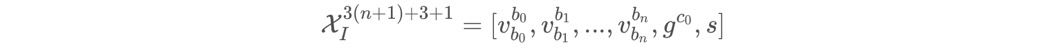这块有个遗留问题，就是为什么要优化速度呢？ 在IMU预积分部分，已经有如下的公式：但是w坐标系我们不知道，只知道c0坐标系，所以需要把上面的公式转到c0坐标系上：上式中，等号左边减去等号右边就是残差，理想状态下，这个残差是0，那么带入上式得：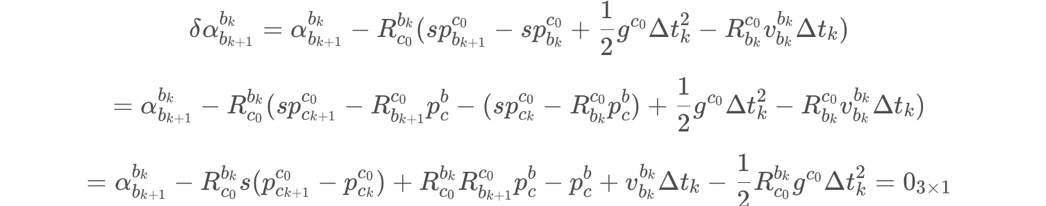把这个 XX = 0的等式也转为 A x = b这种线性方程组的形式，如下式：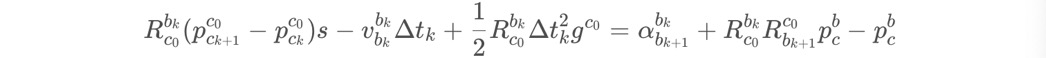或者矩阵的形式：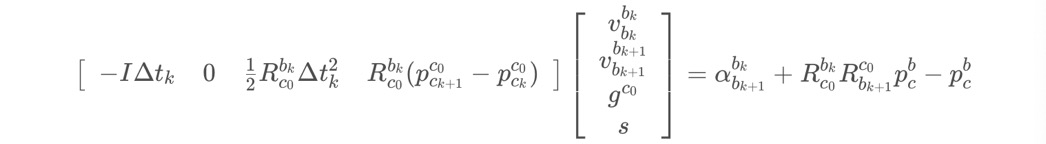对于beta而言，也是类似的，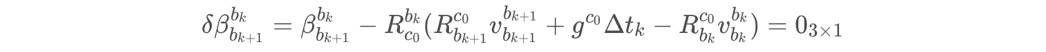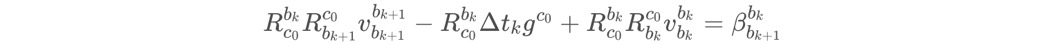那么，把这两个矩阵合体，就能得到论文中的等式：同样采用LDLT分解，就能求出状态量：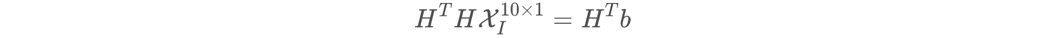接下来是代码解析， (1)参数的传入和容器的定义
bool LinearAlignment(map<double, ImageFrame> &all_image_frame, Vector3d &g, VectorXd &x)
{
int all_frame_count = all_image_frame.size();
int n_state = all_frame_count * 3 + 3 + 1; //需要优化的状态量的个数

MatrixXd A{n_state, n_state};
A.setZero();
VectorXd b{n_state};
b.setZero();

map<double, ImageFrame>::iterator frame_i;
map<double, ImageFrame>::iterator frame_j;


上式中，A和b对应的是Ax=b。注意，传入的参数是all_image_frame，不仅仅是滑窗内的帧。 frame_i和frame_j分别读取all_image_frame中的相邻两帧。 (2)套本小节的最后一个公式，构造Ax=b等式
    int i = 0;
for (frame_i = all_image_frame.begin(); next(frame_i) != all_image_frame.end(); frame_i++, i++)
{
frame_j = next(frame_i);

MatrixXd tmp_A(6, 10);
tmp_A.setZero();
VectorXd tmp_b(6);
tmp_b.setZero();

double dt = frame_j->second.pre_integration->sum_dt;

tmp_A.block<3, 3>(0, 0) = -dt * Matrix3d::Identity();
tmp_A.block<3, 3>(0, 6) = frame_i->second.R.transpose() * dt * dt / 2 * Matrix3d::Identity();
tmp_A.block<3, 1>(0, 9) = frame_i->second.R.transpose() * (frame_j->second.T - frame_i->second.T) / 100.0;
tmp_b.block<3, 1>(0, 0) = frame_j->second.pre_integration->delta_p + frame_i->second.R.transpose() * frame_j->second.R * TIC - TIC;
tmp_A.block<3, 3>(3, 0) = -Matrix3d::Identity();
tmp_A.block<3, 3>(3, 3) = frame_i->second.R.transpose() * frame_j->second.R;
tmp_A.block<3, 3>(3, 6) = frame_i->second.R.transpose() * dt * Matrix3d::Identity();
tmp_b.block<3, 1>(3, 0) = frame_j->second.pre_integration->delta_v;

Matrix<double, 6, 6> cov_inv = Matrix<double, 6, 6>::Zero();
cov_inv.setIdentity();

MatrixXd r_A = tmp_A.transpose() * cov_inv * tmp_A;
VectorXd r_b = tmp_A.transpose() * cov_inv * tmp_b;

A.block<6, 6>(i * 3, i * 3) += r_A.topLeftCorner<6, 6>();
b.segment<6>(i * 3) += r_b.head<6>();

A.bottomRightCorner<4, 4>() += r_A.bottomRightCorner<4, 4>();
b.tail<4>() += r_b.tail<4>();

A.block<6, 4>(i * 3, n_state - 4) += r_A.topRightCorner<6, 4>();
A.block<4, 6>(n_state - 4, i * 3) += r_A.bottomLeftCorner<4, 6>();
}


注意，作者在这里还非常机智地加上了信息矩阵cov_inv。 TODO 但是这些乘1000，除100的操作，最后算起来为啥不等于1呢？ 另外需要注意的地方是，**虽然公式中只计算了相邻2帧，但是代码中，它把所有帧都放进去了，因此，A矩阵和b向量是远大于公式中的尺寸，而且在代码中有一个叠加的操作！非线性优化里构造H矩阵和J矩阵也是一样的。**这里是我看代码前比较疑惑的地方。 (3)ldlt分解，得到尺度和g的初始值，并用先验判断
x = A.ldlt().solve(b);

double s = x(n_state - 1) / 100.0;
g = x.segment<3>(n_state - 4);

if(fabs(g.norm() - G.norm()) > 1.0 || s < 0)
{//如果重力加速度与参考值差太大或者尺度为负则说明计算错误
return false;
}


(4) 利用gw的模长已知这个先验条件进一步优化gc0
    RefineGravity(all_image_frame, g, x);
s = (x.tail<1>())(0) / 100.0;
(x.tail<1>())(0) = s;
ROS_DEBUG_STREAM(" refine     " << g.norm() << " " << g.transpose());
if(s < 0.0 )
return false;
else
return true;
}


见下一节。虽说是优化gc0，实际上都优化了。 6.1.5 利用gw的模长已知这个先验条件进一步优化gc0 但是，这里有一个bug，就是把g当成3自由度的向量优化，但是实际上g只有2自由度，因为它的模长是已知的。所以，在这里，需要想一个办法，就是怎么样用一个2自由度的表达式来表示3维的向量呢？在这里，采用球面坐标进行参数化，也就是用g的模长作为半径画一个半球，上图蓝色线对应的是gc0的测量值的方向(也就是优化前的方向)，在这个交点上找到一个切平面，用gc0，b1，b2构造一个坐标系，那么在轴b1和b2上坐标值w1和w2就是我们需要求的量。求出来之后，把等号右边加在一起，就是优化后的gc0值。 注意，b1的方向是由gc0的测量值的方向与[1,0,0]作叉乘得到的，b2的方向是由gc0的测量值的方向与b1作叉乘得到的。 这样，6.1.4公式得到了更新，此时带优化量减少了1维：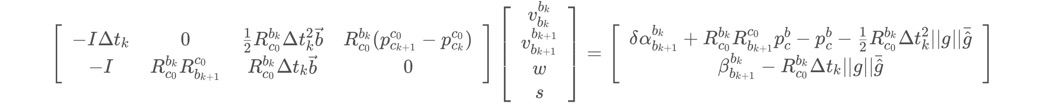即 ，同样使用LDLT分解：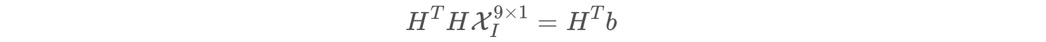其实，在这里，我们可以发现这一部分做的工作和6.1.4是相似的，但是却是在6.1.4的基础上进一步做的工作。 接下来是代码解析， (1)参数的传入和容器的定义
void RefineGravity(map<double, ImageFrame> &all_image_frame, Vector3d &g, VectorXd &x)
{
Vector3d g0 = g.normalized() * G.norm();
Vector3d lx, ly;
//VectorXd x;
int all_frame_count = all_image_frame.size();
int n_state = all_frame_count * 3 + 2 + 1;

MatrixXd A{n_state, n_state};
A.setZero();
VectorXd b{n_state};
b.setZero();

map<double, ImageFrame>::iterator frame_i;
map<double, ImageFrame>::iterator frame_j;


上式中，A和b对应的是Ax=b。注意，传入的参数是all_image_frame，不仅仅是滑窗内的帧。 frame_i和frame_j分别读取all_image_frame中的相邻两帧。 (2)一共迭代四次求解，并构建且向空间
    for(int k = 0; k < 4; k++)
{
MatrixXd lxly(3, 2);
lxly = TangentBasis(g0);
....(3)
}


切向空间的构建如下代码所示：
MatrixXd TangentBasis(Vector3d &g0)
{
Vector3d b, c;
Vector3d a = g0.normalized();
Vector3d tmp(0, 0, 1);
if(a == tmp)
tmp << 1, 0, 0;
b = (tmp - a * (a.transpose() * tmp)).normalized();
c = a.cross(b);
MatrixXd bc(3, 2);
bc.block<3, 1>(0, 0) = b;
bc.block<3, 1>(0, 1) = c;
return bc;
}


(3)套本小节的最后一个公式，构造Ax=b等式
int i = 0;
for (frame_i = all_image_frame.begin(); next(frame_i) != all_image_frame.end(); frame_i++, i++)
{
frame_j = next(frame_i);

MatrixXd tmp_A(6, 9);
tmp_A.setZero();
VectorXd tmp_b(6);
tmp_b.setZero();

double dt = frame_j->second.pre_integration->sum_dt;

tmp_A.block<3, 3>(0, 0) = -dt * Matrix3d::Identity();
tmp_A.block<3, 2>(0, 6) = frame_i->second.R.transpose() * dt * dt / 2 * Matrix3d::Identity() * lxly;
tmp_A.block<3, 1>(0, 8) = frame_i->second.R.transpose() * (frame_j->second.T - frame_i->second.T) / 100.0;
tmp_b.block<3, 1>(0, 0) = frame_j->second.pre_integration->delta_p + frame_i->second.R.transpose() * frame_j->second.R * TIC - TIC - frame_i->second.R.transpose() * dt * dt / 2 * g0;

tmp_A.block<3, 3>(3, 0) = -Matrix3d::Identity();
tmp_A.block<3, 3>(3, 3) = frame_i->second.R.transpose() * frame_j->second.R;
tmp_A.block<3, 2>(3, 6) = frame_i->second.R.transpose() * dt * Matrix3d::Identity() * lxly;
tmp_b.block<3, 1>(3, 0) = frame_j->second.pre_integration->delta_v - frame_i->second.R.transpose() * dt * Matrix3d::Identity() * g0;

Matrix<double, 6, 6> cov_inv = Matrix<double, 6, 6>::Zero();
//cov.block<6, 6>(0, 0) = IMU_cov[i + 1];
//MatrixXd cov_inv = cov.inverse();
cov_inv.setIdentity();

MatrixXd r_A = tmp_A.transpose() * cov_inv * tmp_A;
VectorXd r_b = tmp_A.transpose() * cov_inv * tmp_b;

A.block<6, 6>(i * 3, i * 3) += r_A.topLeftCorner<6, 6>();
b.segment<6>(i * 3) += r_b.head<6>();

A.bottomRightCorner<3, 3>() += r_A.bottomRightCorner<3, 3>();
b.tail<3>() += r_b.tail<3>();

A.block<6, 3>(i * 3, n_state - 3) += r_A.topRightCorner<6, 3>();
A.block<3, 6>(n_state - 3, i * 3) += r_A.bottomLeftCorner<3, 6>();
}


(4)ldlt分解，得到优化后的状态量x
            A = A * 1000.0;
b = b * 1000.0;
x = A.ldlt().solve(b);
VectorXd dg = x.segment<2>(n_state - 3);
g0 = (g0 + lxly * dg).normalized() * G.norm();
//double s = x(n_state - 1);
}
g = g0;
}


6.1.6 利用gc0和gw确定世界坐标系 (1) 找到 c0 到 w 系的旋转矩阵 Rwc0 = exp([θu])gc0已经求出来了，而gw一直就是一个已知的量，因此它们之间的夹角θ是能很快求出来的； 所以我们然后可以用gc0和gw作叉乘得到一个旋转轴u； 最后把c0坐标系，绕着转轴旋转一个θ，就能找到c0 到 w 系的对齐关系，也就是Rwc0 = exp([θu])。 (2) 把所有 c0 坐标系下的变量旋转到 w系下 所有量都乘上Rwc0就可以了。我们定义的c0 与 w 系的原点坐标是重合的。 (3) 把相机平移和特征点尺度恢复到米制单位 初始化大功告成！